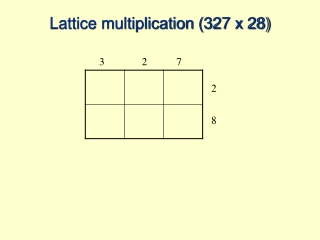DownloadDownload PresentationLattice multiplication (327 x 28)

# Lattice multiplication (327 x 28)

Télécharger la présentation## Lattice multiplication (327 x 28)

- - - - - - - - - - - - - - - - - - - - - - - - - - - E N D - - - - - - - - - - - - - - - - - - - - - - - - - - -
##### Presentation Transcript

1. Lattice multiplication (327 x 28) 3 2 7 2 8

2. Lattice multiplication (327 x 28) 3 2 7 2 8

3. Lattice multiplication (327 x 28) 3 2 7 2 8

4. Lattice multiplication (327 x 28) 3 2 7 2 8

5. Lattice multiplication (327 x 28) 3 2 7 2 8

6. Lattice multiplication (327 x 28) 3 2 7 2 8

7. Lattice multiplication (327 x 28) 3 2 7 2 8

8. Lattice multiplication (327 x 28) 3 2 7 2 8

9. Lattice multiplication (327 x 28) Now add the diagonals

10. Lattice multiplication (327 x 28) 6 Now add the diagonals

11. Lattice multiplication (327 x 28) 6 6+5+4 = 15

12. Lattice multiplication (327 x 28) 1 5 6 1+1+4+1+4=11

13. Lattice multiplication (327 x 28) 1 1 1 5 6

14. Lattice multiplication (327 x 28) 1 1 1 5 6 1+6+2=9

15. Lattice multiplication (327 x 28) 1 1 9 1 5 6

16. Lattice multiplication (327 x 28) 1 1 0 9 1 5 6

17. Lattice multiplication (327 x 28) 1 1 0 9 1 5 6 Now read off the digits according to the diagonal place value columns

18. Lattice multiplication (327 x 28) 1 1 0 9 1 5 6 6 is in the ones column 5 in the tens 1 in the hundreds 9 in the thousands 0 in the ten-thousands

19. Lattice multiplication (327 x 28) 1 1 0 9 1 5 6 6 is in the ones column 5 in the tens 1 in the hundreds 9 in the thousands 0 in the ten-thousands So 327 x 28 = 9156

20. Lattice multiplication (327 x 28) 1 1 0 9 1 5 6 Now calculate 327 x 28 by another method. Can you see how your method is similar to this one?

21. Lattice multiplication (327 x 28) 327 x 28 56 160 2400 140 400 6000 9156 1 1 0 9 1 5 6 Did you write out all 6 partial products separately? (like this)

22. Lattice multiplication (327 x 28) 327 x 28 56 160 2400 140 400 6000 9156 327 x 28 2616 6540 9156 1 1 0 9 1 5 6 Did you write out all 6 partial products separately? (like this) Or did you use a condensed version? (like this)

23. Lattice multiplication (327 x 28) 327 x 28 56 160 2400 140 400 6000 9156 327 x 28 2616 6540 9156 1 1 0 9 1 5 6 Did you write out all 6 partial products separately? (like this) Or did you use a condensed version? (like this) Challenge: see if you can find each of the 6 partial products in the lattice multiplication!